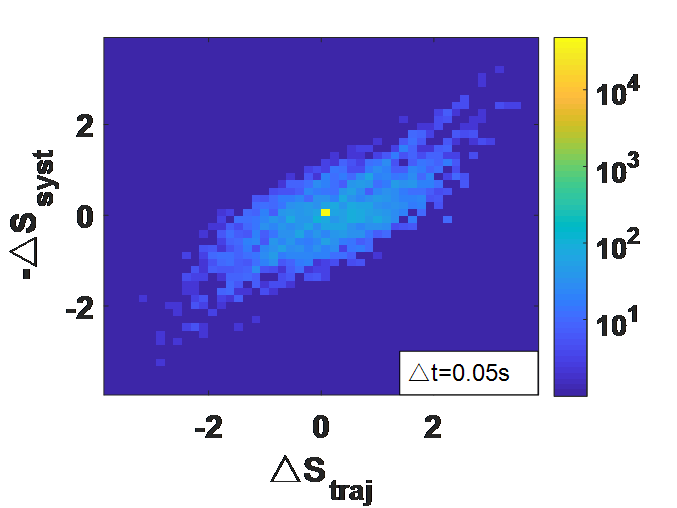• 2022-04-19 09:25:16

# matlab中的对数使用

Matlab中的对数使用，matlab中提供了log10、log2和以e为底的对数。

1、以e为底的对数用log()表示，如log(a)。

2、以10为底的对数用log10()表示，如log10(a)。

3、以2为底的对数用log2()表示，如log2(a)。

4、对于其它如 log ⁡ a b \log a^b ，用logb/loga表示。

更多相关内容
• 原来要代表颜色的数bai据以10为底的对数： log10() 然后用下面语句标注： colorbar('YTick',[-6,-5,-4,-3,-2,-1,-0],... 'YTickLabel',{'1E-6','1E-5','1E-4','1E-3',... '1E-2','1E-1','1E0'}); 实际上，数据...

原来要代表颜色的数bai据取以10为底的对数：log10()

然后用下面语句标注：
colorbar('YTick',[-6,-5,-4,-3,-2,-1,-0],...
'YTickLabel',{'1E-6','1E-5','1E-4','1E-3',...
'1E-2','1E-1','1E0'});

实际上，数据取了对数之后标注的位置是 -6~0
只是用'YTickLabel'，让其在对应位置显示需要的文本[N,Xedges,Yedges] = histcounts2(entropy(:,1),entropy(:,2),[50,50]);
max1=max(entropy(:,1))
max2=max(entropy(:,2))
min1=min(entropy(:,1))
min2=min(entropy(:,2))

%N=fliplr(N);
figure(1);
N1=flipud(rot90(N));       %N逆时针旋转90度为想要的矩阵，上下翻转主要是保证后面纵坐标正向
%N2=N1./max(max(N));       %归一化

%% ------对于数据分布很不均匀的，可以用log()
logN1=log10(N1);
logN1(isinf(logN1))=0;    %搜索为Inf的数据

imagesc([min1,max1],[min2,max2],log10(N1));
%imagesc([min1,max1],[min2,max2],N1,[0,1000]);
set(gca,'YDir','normal');        %由于imagesc纵坐标轴默认为反向，所以需要改为正方向
h=colorbar;
set(h,'YTick',[1,2,3,4]','YTicklabel',{'10^1','10^2','10^3','10^4'})   %实现colorbar的对数显示
xlabel('△S_{traj}','Fontweight','bold','FontSize',20);
ylabel('-△S_{syst}','Fontweight','bold','FontSize',20);
set(gca,'FontSize',20,'Fontweight','bold');   %调节坐标轴字体大小粗细
annotation('textbox',[0.7 0.01 0.1 0.1],'Linestyle','-','String','△t=0.05s')

展开全文colorbar
• 本教程分享：《matlab对数函数》，MATLAB 中如何输入 对数函数方法/步骤1、自然数对数 log(x)我们在MATLAB主窗口中输入a1=log(2.7183)，回车，我们可以看到a1近似为1，e约等于2.7183,2、以2为底数的对数 log2(x)我们...

本教程分享：《matlab对数函数》，

MATLAB 中如何输入 对数函数

方法/步骤

1、自然数对数 log(x)

我们在MATLAB主窗口中输入a1=log(2.7183)，回车，我们可以看到a1近似为1，e约等于2.7183,

2、以2为底数的对数 log2(x)

我们在MATLAB主窗口中输入a2=log2(4) ，回车，可以看到结果a2=2

3、以10为底数的对数 log10(x)

我们在MATLAB主窗口中输入a3=log10(10) ，回车，可以看到结果a3=1

4、其他底数对数logM(N)

这种对数需要进行一个简单的中间变换，logM(N)=log(N)/log(M)，这样写方便，用log10() 以及log2()都可以。我们在MATLAB主窗口中输入如下命令：

a4=log(64)/log(8) 回车

我们可以看到 ，以8为底64的对数为2,

在线等。matlab上的对数函数数据拟合。y=algx+b

实验数据：

x=[500 550 600 650 700 750 800 850 900 950 1000];

y=[62.4 69.2 75.4 82.2 70.4 68.4 75.2 77.8 71.6 75.6 72.2];

图中既有曲线也有数据点，最好能求出a,b.

x=[500 550 600 650 700 750 800 850 900 950 1000];

y=[62.4 69.2 75.4 82.2 70.4 68.4 75.2 77.8 71.6 75.6 72.2];

f = fittype('a*log10(x)+b'); % 拟合函数的形式

fit1 = fit(x',y',f,'StartPoint',[x(1) y(1)]);

a = fit1.a; % a的值

b = fit1.b; % b的值

fdata = feval(fit1,x'); % 用拟合函数来计算y

figure

plot(x,y); hold on

plot(x,fdata','r'); hold off

legend('Ori data',' Fitting data');

更多追问追答

追问

能求出a,b值吗？

追答

老大，里面不是有a, b值么，我还做了注释！

追问

哦，知道了。你能尽量多加点注释吗，我是matlab菜鸟。

追答

哦，知道了，你还需要加什么注释么？

追问

以下两句没有注释，看不懂。

fit1 = fit(x',y',f,'StartPoint',[x(1) y(1)]);

legend('Ori data',' Fitting data');

再提问就得扣分了。。。

下面的程序跟你的出图一样，但好像简单些

clc;clear;

x=[500 550 600 650 700 750 800 850 900 950 1000];

y=[62.4 69.2 75.4 82.2 70.4 68.4 75.2 77.8 71.6 75.6 72.2];

plot(x,y);

x_log=log10(x);

A=polyfit(x_log,y,1)

hold on;

plot(x,A(1).*log10(x)+A(2),'r');

追答

fit(x',y',f,'StartPoint',[x(1) y(1)]) 的意思， 是生成一个拟合函数，用的数据是x, y, 注意x'是要将x写成一个n-by-1的向量，y也如此。所以里面是fit(x',y',...). f 是上面定义的拟合函数的形式。'StartPoint'是起始点，定义的起始点x(1),y(1).

legend('Ori data',' Fitting data'); 就是标注两条曲线，第一个是原始曲线，第二个是拟合后的曲线

另一个人用polyfit,这个只能用于多项式拟合。其余的都不行了，我的这个，什么形式的都可以

追问

最后一个问题，为什么要x,y都要转置成列向量？

追答

恩，这个是matlab 自带函数fit所要求的。 fit(x,y,f...)中的x, y必须是要列向量，否则会报错。

在matlab中怎样表示ln？

MatLab中ln 就是log(),

以10为底的对数用log10()

一般地，对数函数以幂(真数)为自变量，指数为因变量，底数为常量的函数。

对数函数是6类基本初等函数之一。其中对数的定义：

如果ax=N(a>0，且a≠1)，那么数x叫做以a为底N的对数，记作x=logaN，读作以a为底N的对数，其中a叫做对数的底数，N叫做真数。

一般地，函数y=logax(a>0，且a≠1)叫做对数函数，也就是说以幂(真数)为自变量，指数为因变量，底数为常量的函数，叫对数函数。

其中x是自变量，函数的定义域是(0，+∞)，即x>0。它实际上就是指数函数的反函数，可表示为x=ay。因此指数函数里对于a的规定，同样适用于对数函数。

在MATLAB中对数如何表示

log(x)：以e为底的对数，即自然对数

log2(x)：以2为底的对数

log10(x)：以10为底的对数

如何在matlab中求对数？

1、第一步首先介绍自然数对数log(x)，电脑中打开matlab之后，在命令行窗口输入a=log(2.7183),按回车键后，可以看到结果近似为1，e的值近似为2.7183，

2、第二步介绍以2为底的对数函数log2(x)，在命令行窗口中输入b=log2(8)，按回车键，可以看到b=3

3、第三步介绍以10为底的对数函数log10(x)，在命令行窗口中输入c=log10(1000)，按回车键，可以看到c=3，

4、第四步介绍其它的对数函数logX(Y)，这种对数函数要转换成logX(Y)=log(Y)/log(X)格式，在命令行窗口输入d=log(9)/log(3),按回车键，可以看到d的结果为2，

5、第五步我们在matlab的工作区中，可以看到存储的变量结果

matlab中ln函数怎么表示

用log()函数

例如log(exp(1))

输出

1

--------------------------------

注：以2为底的对数函数为log2()，以10为底的对数函数为log10()，其他数为底的对数函数可用换底公式求得

请问matlab怎么编辑任意底数的指数函数和对数函数？

注意取值范围,定义域还有题本生的隐含条件

MATLAB中的自然对数e，是怎么表示的

自然对数是log()函数

自然对数的底数e，也就是自然指数函数exp(x),当x取1时候的值

所以用exp(1)可以获得

用matlab描述以0.5为底的对数图像

网上都是大于一的对数图像，然后用换底公式做出来的对数图像没有0

x=0:1;

y=log(x)/log(1/2);

plot(x,y)

可这样：

x=0:0.01:1;

y=log(x)/log(1/2);

plot(x,y)

matlab拟合对数函数，怎么弄

差距太大了...

差距太大了k

m

matlab拟合对数函数，可以这样来做：

x=[。。。]; y=[。。。]; %已知数据

func=@(a,x)a(1)*log(a(2)*x^4+a(3)*x^3+a(4)*x^2+a(5)*x+a(6))/log(3) %根据拟合精度，可以调整

a0=[0,0,0,0,0,0]; %初值，可以调整

[a,r] = nlinfit(x,y,func,a0) %a拟合系数，r差值

当r比较小(接近于零)，说明拟合结果是合理的

有数据吗？如有困难可以通过私信或其他方式帮助你。

展开全文• ## Matlab的对数

千次阅读 2021-04-23 21:51:31
但是在matlab中，log默认为e的对数，而如果需要其他的对数，则是log10，log2等等；例如：log10(10)=1等等。rand - Uniformly distributed pseudorandom numbersThis MATLAB function returns a pseudorandom s...

数学中的lg表示是以10为底的对数，而自然对数表示为ln，也就是以e为底数的对数；但是在matlab中，log默认为e的对数，而如果需要其他的对数，则是log10，log2等等；例如：log10(10)=1等等。

rand - Uniformly distributed pseudorandom numbers

This MATLAB function returns a pseudorandom scalar drawn from the standard

uniform distribution on the open interval (0,1)

这里表示生成的数据是(0,1)之间的均匀分布的数值；

sign - Signum function

This MATLAB function returns an array Y the same size as X, where each element

of Y is:

Y = sign(X)

对于符号函数，在matlab中，不是使用sgn这种形式，而是使用sign的命令；

norm - Vector and matrix norms

This MATLAB function returns the 2-norm of input X and is equivalent to

norm(X,2).

n = norm(X)

n = norm(X,p)

对于矩阵以及向量的范数，其默认的情况下是表示二范数；

nargin - Number of function input arguments

This MATLAB function returns the number of input arguments passed in the call to

the currently executing function.

表示输入的变量个数；

同时这里需要指出matlab中和C语言中的switch命令的区别，其对于matlab来说，如下的命令：

input_num=input('Enter the number:');

switch input_num

case -1

disp('negative num');

disp('minus one');

case 0

disp('zero');

case 1

disp('positive num');

otherwise

disp('other number');

end

是正确的，而且其能够直接跳出，例如输入-1的时候，其不会显示zero等下面的输出语句；但是对于C语言来说，其正确的应该是：

switch(input_num)

{

case -1

disp('negative num'); disp('minus one'); break;

case 0

disp('zero');break;

case 1

disp('positive num');break;

default

disp('other number');break;(这个可以加也可以不加)

}(这个也可以不加)

这样才和matlab是一致的。主要是break那里的区别。

print - Print figure or save to file

This MATLAB function sends the contents of the current figure, including bitmap

representations of any user interface controls, to the printer using the device

and system printing command defined by printopt.

print

print('argument1','argument2',...)

print(handle,'filename')

print argument1 argument2 ... argumentn

这里主要是表示对图形的输出；

fprintf - Write data to text file

This MATLAB function applies the formatSpec to all elements of arrays A1,...An

in column order, and writes the data to a text file.

fprintf(fileID,formatSpec,A1,...,An)

fprintf(formatSpec,A1,...,An)

nbytes = fprintf(___)

这里主要是对于数据的输出；

input_num=input('Enter the number:');

if input_num>0

display('positive number');

fprintf('input_num=%f\n',input_num);

elseif input_num==0

display('zero');

fprintf('input_num=%f\n',input_num);

else input_num<0

display('negative number');

fprintf('input_num=%f\n',input_num);

end

注意可以有多个elseif语句，但是只能拥有一个if和end语句；并且disp以及display都可以使用。

对于循环来说，while循环的执行次数是不确定的，但是for循环所执行的次数是一致的。

展开全文• 用于设置色标表示形式，colorscale有两种表现形式，‘Linear’ or ‘log’. clear;close; colormap(jet(50)); h = figure(1);set(h,'position',[10 10 1600 600]) subplot 121 mesh(peaks);colorbar;......开发语言 图像处理
• 画图时有时不想使用自然坐标（线性刻度），而需要使用对数坐标，此处给出几种方法：1使用ezplot画图时，坐标用对数坐标。 ezplot默认横坐标从-2pi到2pi 举例：以y=x为例 a) 其中一方为对数坐标，另一方为线性坐标 ...
• Matlab R2014b以来,事情变得非常简单.不需要文件交换的附加功能,只需要一些undocumented features.基本思想是获取标记的隐藏句柄并应用值< 1为EdgeColorData中的最后一个值,以实现所需的透明度. 开始了：%// ...
• When using MATLAB to calculateLog_likelihood=log((1/1e8)*exp(-0.5*SSR)),if SSR (i.e. standard squared error) is large (e.g. SSR=1e4) then the exp() becomes zero and the Log_likelihood becomes -inf.Is ...
• 函数是log10()，如求10的对数，为log10(10)=1。注意不是log!（这个是以e为底数的对数
• 后来看了这个例子发现不是X维数的原因，其原因是对数函数ln(x)的x值要大于0，我的数据算出来均为负数，所以才出现了这样的情况。所以我将x改为绝对值的形式，就避免了这种情况。并且关于optimset函数，是一个优化...
• MATLAB中如何在对数坐标、极坐标、双轴坐标中绘图，以及极坐标与直角坐标的转换
• MATLAB中输入对数函数主要分为以下两种类型： 一、直接型 以e、2或者是10为底的对数的话，直接输入：y=log(x)，y=log2(x)，y=log10(x)。例如，a1=log(2.7183)；a2=log2(2)；a3=log10(10)，其结果如下图： 二、转换...
• ## matlab对数计算方法

千次阅读 2020-07-10 11:58:39windows
• 对数函数运算法则是什么呢？●中文名对数函数外文名LogarithmicFunction别称对函数表达式ylogax(a0a≠1)提出者约翰·纳皮尔提出时间16世纪末应用学科数学适用领域范围解析几何适用领域范围代数学自然科学函数最值无...
• 利用Matlab提取图片中曲线数据前一段时间看到一篇文章“利用Matlab提取图图片中的数据”，觉得思路挺好，遂下载下来研究了一番，发现作者所编写的程序没有考虑原始图片非水平放置的情况，而实际扫描图片时，将图片...
• 文档中包含网易云课堂MATLAB教程中 冒号区间裁剪，冒号等差序列，删除整行整列的操作，简单易学，内容详细。
• Matlab中怎么表示自然对数？&gt&gtlog(10)ans=2.3026&gt&gtlog10(10)ans=1&gt&gthelplogLOGNaturallogarithm.LOG文件(十) 地峡如果x.complex resultsareproductedifx元素不为正....
• 灰度对数变换公式如下：                              &...算法 计算机视觉
• matlab有semilogx,semilogy和loglog三种针对一维数据的双对数坐标显示。对于二维数据应该任何操作呢？ 代码 subplot(121) contourf(peaks,30,'lines','none') colormap jet axis equal subplot(122) contourf(peaks...
• 该练习将 -1 和 +1 之间的语音电平间隔划分为 2B 个均匀间隔（对于均匀量化器）和 2B 个伪对数间隔（对于 mu-law 量化器）。 每个量化间隔的确切位置决于量化是使用中上升间隔还是中踏间隔，还决于量化是使用舍...
• 文章目录一、坐标系介绍1.1 算数坐标系（笛卡尔坐标系）1.2 对数坐标系1.3 两者的区别二、基于matlab的实例分析2.1 举例2.2 分析与证明 一、坐标系介绍 1.1 算数坐标系（笛卡尔坐标系） 算术坐标系统是普通的笛卡儿...
• 由于很多数据的变量间存在级别差异，直接比较两者的变化会不如人意。对数据的不同变量进行相对值变换，可以消除不同变量的量级问题。
• 文章目录 前言 方法1 方法2 gca 用法 举例 set XScale 前言 当我使用MATLAB画了一幅曲线图之后，例如： 由于x轴10以下的单位内，y值比较小，而之后比较大，因此，我想更清晰地看y值得变化趋势，因此我想用对数标准的...
• 然后尝试这些值的对数并改为对对数进行运算。 在这种情况下：log (p • q) = log p + log q 很容易计算，但问题是从 log a 和 log 的值计算（或近似）log (a + b) 的值湾 让我们假设 log a 和 log b 是已知的，...
• ln5是以什么为底的对数ln5是以(e)为底的对数 ，loge 5=ln5朋友，请采纳正确答案，你们只提问，不采纳正确答案，回答都没有劲！！！朋友，请【采纳答案】，您的采纳是我答题的动力，如.公式：指数函数a^x的导数是a^x*...
• 1. 均匀分布 函数形式： x=rand(n,m) n - 行数 ...3. 对数正态分布 函数形式 x=lognrnd(mu,sigma,a,b) mu- 对数值的均值（mean of logarithmic values）；mu = log((m^2)/sqrt(v+m^2)); （m - ..开发语言
• 该练习将 -1 和 +1 之间的语音电平间隔划分为 2B 个均匀间隔（对于均匀量化器）和 2B 个伪对数间隔（对于 mu-law 量化器）。 每个量化间隔的确切位置决于量化使用的是中间上升间隔还是中间踏步间隔，还决于量化...
• 对数图形的绘制：x = logspace(-1,1,100);%在10^(-1)到10^(1)中100个值y = x.^2;subplot(2,2,1); %用线性的方式画图plot(x,y);title('Plot');subplot(2,2,2);semilogx(x,y);%x轴log (10^-1、10^0、10^1等间隔)......

# matlab取对数matlab 订阅